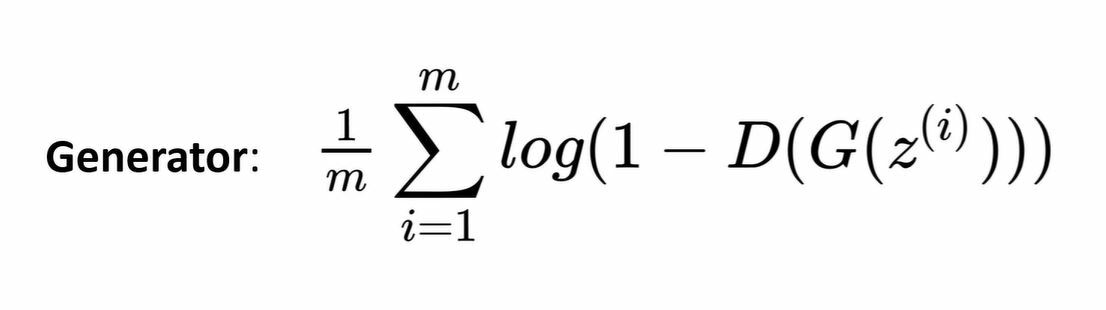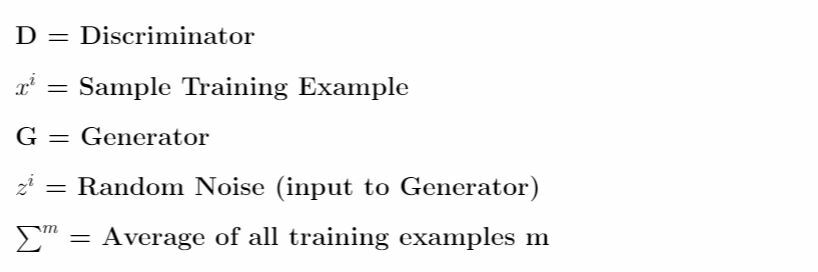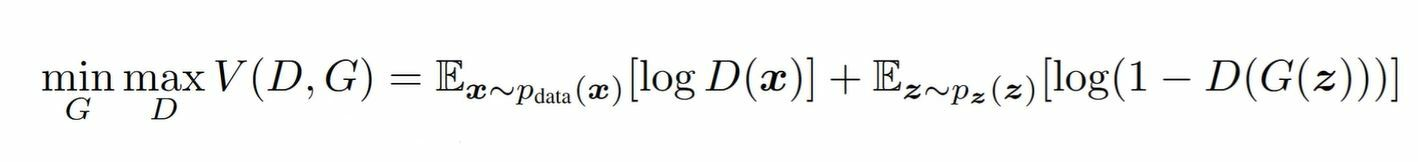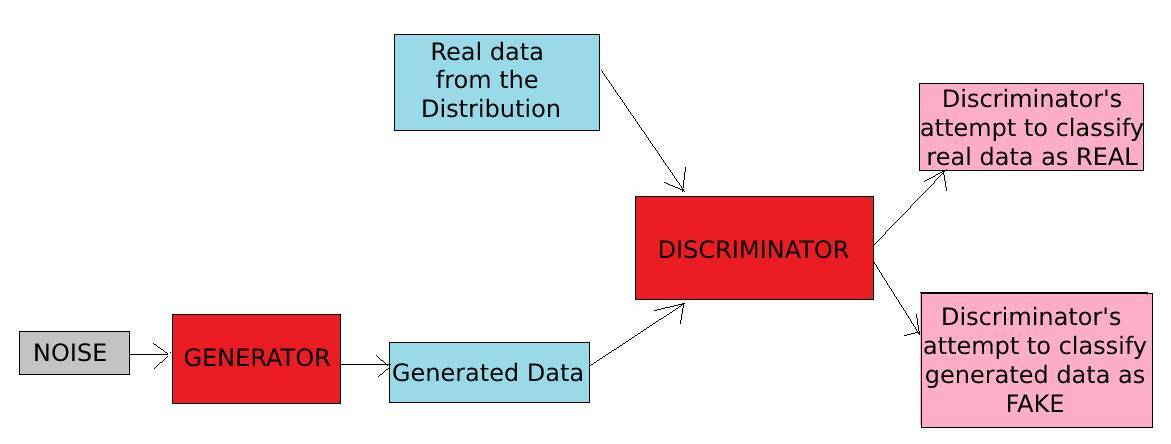# .css-df1pn7{display:block;width:16rem;}## IntroductionPublished on Jun 13, 2021

Subscribe to my newsletter and never miss my upcoming articles

Ever wondered how Mona Lisa would have looked in real life?Or have you ever wanted to create new faces so well that most people can’t distinguish the faces it generates from real photos?How would you feel if I would say that you can predict future frames of a video?Fascinated, right?

Each of these is possible with the power of GANs. Let's understand what these are and how they work!

Generative Adversarial Network or GANs are deep generative models. These are a combination of two networks that are opposed against each other and are neural network architectures that are capable of generating new data.So let us break down the word and understand them. `Generative` means capable of production or reproduction, `Adversarial` means two sides who oppose each other and `Network` means a system of interconnected things.

GANs are actually two different networks joined together and are composed of two halves:

1. Generator
2. Discriminator

But before understanding what these two are, let us know what is Loss Function 🤔.

### Loss Function:

The loss function describes how far the results produced by our network are from the expected result: how far an estimated value is from its true value. Its objective isn't to make the model good but is to keep it from going wrong. Loss Function gives us the direction of the optimal solution.

## Generator:

The generator is the neural network architecture that takes in some input and reshapes it to get a recognizable structure that is close to the target. The main aim of the generator is to make the output look as close as possible to the real data.But to make this possible, the generator network needs to be trained heavily.

#### Let's understand how Loss Function for Generator Network works:We want to fool the Discriminator into believing that the output from the Generator is actually real.

So this term is going to be 1 if we are successfully able to fool the Discriminator. Hence, we will have a log(something close to zero).

So does the generator want to maximize or minimize the loss?

Generator wants to minimize this loss.

## Discriminator:

The discriminator is a regular neural network architecture that does the classification job to categorize real data from the fake samples generated by the Generator.Discriminator's training data comes from two sources:

1. Read data used for training.
2. Fake data generated by the Generator.

#### Let's understand how Loss Function Discriminator Network works:Here,Let's divide this equation into 2 terms:

First term: We take the log of D(x(i)), where `x(i) = real` so we want our `Discriminator` to output `1` here. So, if we look at `log(1)`, the output is going to be `zero`.

Second term: `log(1-D(G(z)))` generator is going to take in some random noise and it's gonna output something close to real(close to reality) and the discriminator is going to output either `0 or 1` and from discriminator's point of view, we want the output to be `zero` here.

So does the Discriminator want to maximize or minimize the loss?

Discriminator wants to maximize this loss.

## Combining both of these:LHS of this expression means that we want to minimize w.r.t Generator and maximize w.r.t Discriminator for some value function V takes to input the Generator and the Discriminator(D, G).

In practice the generator is trained to instead:to maximize the Generator MaxG because this new expression leads to non-saturating gradients which makes it a lot easier for training.

In simple words, the generative and discriminator models play a symmetric opponent game or a zero-sum game with each other that is where one side's benefits come at the expense of the other.In the end, after a lot of training process, the generator can make indistinguishable things from real ones and the Discriminator is forced to Guess.Both the generator and the discriminator start from scratch without any prior knowledge and are simultaneously trained together.

`Generative models can generate new examples from the sample that are not only similar to the class but real.`

## Wondering what are GANs used for?

GANs have seen major success in the past years. They have a wider range of applications. Usage of GANs is not limited to these. Here are a few use cases.

1. Generating New Data: Rather than augmenting the data, new training data samples can be generated by GANs from the existing data. Here is an example of Fashion MNIST samples generated by GANs.2. Super Resolution: GANs can be used to enhance the resolution of images and videos. Here is an example of video super-resolution done by tempoGAN.3. Security: GANs can be used for malware detection and intrusion detection. GANs can be used in a variety of cybersecurity applications, including enhancing existing attacks beyond what a standard detection system can handle.

4. Audio Generation: GANs can be used to generate high-quality audio, instrumentals, and voice samples. MuseGAN and [WaveGAN] are two such GANS.5. Healthcare: GANs can be utilized to detect tumors. By comparing photos with a library of datasets of healthy organs, the neural network can be used to identify cancers. By finding disparities between the patient's scans and photos and the dataset images, the network can discover abnormalities in the patient's scans and photographs. Using generative adversarial networks, malignant tumors can be detected faster and more accurately.1. Original GAN Paper - Ian Goodfellow